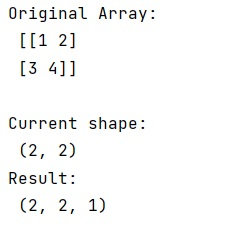# How to make a 2d NumPy array a 3d array?

Learn, how to make a 2d NumPy array a 3d array in Python?
Submitted by Pranit Sharma, on February 26, 2023

NumPy is an abbreviated form of Numerical Python. It is used for different types of scientific operations in python. Numpy is a vast library in python which is used for almost every kind of scientific or mathematical operation. It is itself an array which is a collection of various methods and functions for processing the arrays.

## Making a 2d NumPy array a 3d array

Suppose that we are given a 2d array with shape (x, y) which we want to convert to a 3d array with shape (x, y, 1).

When we convert a 2d array into a 3d array, we are just adding one more axis or we are just modifying the shape of the array.

For this purpose, we always use numpy.newaxis() to add a new axis or to increase the dimension of the numpy array. If we are given a numpy array of shape (x,y), newaxis will add 1 more axis and the shape will become (x,y,1).

Let us understand with the help of an example,

## Python code to make a 2d NumPy array a 3d array

```# Import numpy
import numpy as np

# Creating a numpy array
arr = np.array([[1,2],[3,4]])

# Display original array
print("Original Array:\n",arr,"\n")

# Display current shape
print("Current shape:\n",arr.shape)

# Adding a new axis and making
# the array as a 3d array
res = arr[:, :, np.newaxis]

# Display resultant array shape
print("Result:\n",res.shape)
```

Output:Languages: » C » C++ » C++ STL » Java » Data Structure » C#.Net » Android » Kotlin » SQL
Web Technologies: » PHP » Python » JavaScript » CSS » Ajax » Node.js » Web programming/HTML
Solved programs: » C » C++ » DS » Java » C#
Aptitude que. & ans.: » C » C++ » Java » DBMS
Interview que. & ans.: » C » Embedded C » Java » SEO » HR
CS Subjects: » CS Basics » O.S. » Networks » DBMS » Embedded Systems » Cloud Computing
» Machine learning » CS Organizations » Linux » DOS
More: » Articles » Puzzles » News/Updates